Amalgam of groups

A family of groups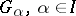, that satisfies the condition that the intersection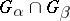is a subgroup inand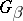for any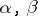from. An example of an amalgam of groups is an arbitrary family of subgroups of a given group. An imbedding of an amalgam of groups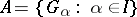into a groupis a one-to-one mapping of the union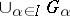intowhose restriction to eachis an isomorphism. An amalgam of groups in which all intersectionsare identical (and equal to, say, a subgroup) is imbeddable in the group that is the free product of the groups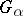with the amalgamated subgroup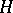. On the other hand, there exists an amalgam of four Abelian groups that is not imbeddable in a group. The principal problem concerning amalgams of groups is, generally speaking, as follows. Letbe possible properties of groups. The question to be answered is the nature of the conditions under which an amalgam of groups with the propertyis imbeddable in a group with the property. It was found that all amalgams of two finite groups are imbeddable in a finite group. An amalgam of three Abelian groups is imbeddable in an Abelian group. An amalgam of four Abelian groups imbedded in a group is contained in an Abelian group. There exists an amalgam of five Abelian groups which is imbeddable in a group, but not in an Abelian group. Another problem that has been studied is the imbeddability of an amalgam of groups ifdenote solvability, nilpotency, periodicity, local finiteness, etc. (in different combinations).

In the definition of amalgam above, think of theas all being subsets of some large set. The amalgamated product of groups"over a common subgroup U" is constructed as follows. Let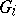be a set of groups indexed by the set. For eachletbe a subgroup ofand for eachlet there be an isomorphism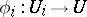identifying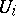and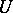. Consider the set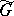of all words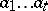with each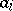from some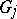, and consider the following elementary equivalences

1) if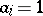then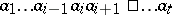is equivalent to;

2) ifandbelong to the same groupandinthen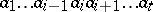is equivalent to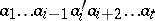;

3) ifand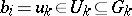and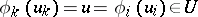, thenis equivalent to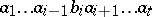.

Letbe the equivalence relation generated by these elementary equivalences, thenis the amalgamated product of the, more precisely of the(i.e. the free product with amalgamated subgroup of the); the group law is induced by concatenation.

Amalgamated products are non-trivial. This follows from the following canonical form theorem. For eachselect a setof left coset representatives ofin. Then each word is equivalent to precisely one of the formwith eachin some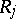,andand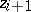belonging to different's for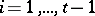. If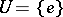one obtains of course the free product of the. A subgroup of a free product is itself a free product (Kurosh' theorem). Subgroups of a product with an amalgamated subgroup need not be themselves of this type. The reason is that ifis the amalgamated subgroup, then one can take subgroups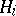of thewith different intersections withso that thewill amalgamate in various different ways. This leads to generalized amalgamated products and the notion of amalgam as defined above. The theory of these is still incomplete.